Matrix rings: In view of Proposition 2.6, the definition of the product of two n×n matrices now
makes sense: AB = D, where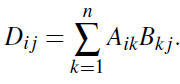So we are in the position to prove Proposition 2.1. A complete proof of this proposition involves verifying all the ring axioms. The arguments are somewhat repetitive; I will give proofs of two of the axioms.
Axiom (A2): Let 0 be the zero element of the ring R, and let O be the zero matrix in Mn(R), satisfying Oi j = 0 for all i, j. Then O is the zero element of
Mn(R): for, given any matrix A,
(O A)i j = Oi j Ai j = 0 Ai j = Ai j, (A O)i j = Ai j Oi j = Ai j 0 = Ai j,
using the properties of 0 2 R. So O A = A O = A. Axiom (D): the (i, j) entry of A(B C) is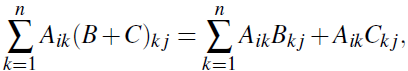by the distributive law in R; and the (i, j) entry of AB AC is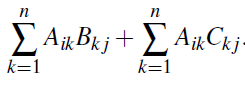Why are these two expressions the same? Let us consider the case n = 2. The first expression is
Ai1B1 j Ai1C1 j Ai2B2 j Ai2C2 j,
while the second is
Ai1B1 j Ai2B2 j Ai1C1 j Ai2C2 j.
(By Proposition 2.6, the bracketing is not significant.) Now the commutative law for addition allows us to swap the second and third terms of the sum; so the two
expressions are equal. Hence A(B C) = AB AC for any matrices A,B,C. For n>2, things are similar, but the rearrangement required is a bit more complicated. The proof of the other distributive law is similar.
Observe what happens in this proof: we use properties of the ring R to deduce properties of Mn(R). To prove the distributive law for Mn(R), we needed the distributive law and the associative and commutative laws for addition in R. Similar things happen for the other axioms.

Polynomial rings: What exactly is a polynomial? We deferred this question before, but now is the time to face it.
A polynomial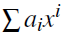is completely determined by the sequence of its coefficients a0,a1, . . .. These have the property that only a finite number of terms in the
sequence are non-zero, but we cannot say in advance how many. So we make the following definition:
A polynomial over a ring R is an infinite sequence (ai)i0 = (a0,a1, . . .)
of elements of R, having the property that only finitely many terms are non-zero; that is, there exists an n such that ai = 0 for all i > n. If an is the last non-zero
term, we say that the degree of the polynomial is n. (Note that, according to this definition, the all-zero sequence does not have a degree.) Now the rules for addition and multiplication are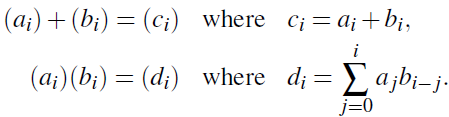Again, the sum in the definition of multiplication is justified by Proposition 2.6. We think of the polynomial (ai)i0 of degree n as what we usually write as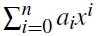; the rules we gave agree with the usual ones. Now we can prove Proposition 2.2, asserting that the set of polynomials over a
ring R is a ring. As for matrices, we have to check all the axioms, which involves a certain amount of tedium. The zero polynomial required by (A2) is the all-zero sequence. Here is a proof of (M1). You will see that it involves careful work with dummy subscripts!
We have to prove the associative law for multiplication. So suppose that f = (ai), g = (bi) and h = (ci). Then the ith term of f g is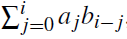j=0 ajbi−j, and so the ith term of ( f g)h is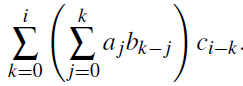Similarly the ith term of f (gh) is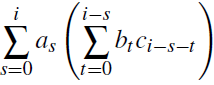.
Each term on both sides has the form apbqcr, where p,q, r  0 and p q r = i. (In the first expression, p = j, q = k− j, r = i−k; in the second, p = s, q = t, r = i−s−t.) So the two expressions contain the same terms in a different order. By the associative and commutative laws for addition, they are equal.